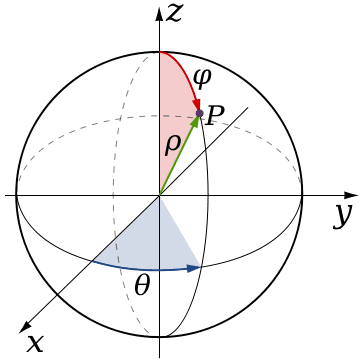# How do i prove the volume of a sphere by double integration?

using the technique of double integration i need to prove that the volume of a sphere =
4/3*Pi*r^3....i kinda have some idea but dont know what original function to start the double integration on?

Help!

Thank you...

arildno
Homework Helper
Gold Member
Dearly Missed
A couple of alternatives:

A) Disk method:
Think of slicing the ball in horizontally placed disks about the vertical, where the vertical variable is called z

Use the Pythagorean theorem to derive the local radii as a function of z, and integrate the area function for the disks from -r to r.

B) Shell method:
Think of the sphere as consisting of concentric spherical SHELLS, with radii varying from 0 to r.
Find the area function for the shells as a function of the radial variable, and integrate.

It says i need to use the multiple integration texhnique....

What's the equation for a sphere? Integrate that function around a circular region of some radius, r.

I dont know, iv found a few different equations for a sphere and not sure which one to believe...or use! one was x^2+y^2+z^2 = r^2
another one used sin(theta)...

Both of the equations you mentioned can be used.

Say you have a given sphere in R3, centered at the origin (0,0,0).

I am going to use spherical coordinates, as it is a lot easier to calculate the volume of a sphere with spherical coordinates.

Basically, if you integrate teh function 1 over a volume, you get the volume of the object.

EDIT: thed function you integrate over is 1 in normal coordinate system, but when yoiu go to spherical coordinates you have to multiply the function 1 by the Jacobian, rho^2 sin(phi). So the integral is tripple integral of rho^2 sin(phi), according to the following diagram and limits. you are integrating over Rho, phi, and theta:Limits:
Rho: <0,r>
Phi: <-pi/2 , pi/2>
Theta: <0 , 2pi>

This will yield the volume using a Tripple integral.

For some reason the Latex code isnt working right now.

$$\int ^{0}_{r} \int ^{-\pi / 2}_{\pi / 2} \int ^{0}_{2\pi} \rho ^{2} sin(\phi ) d\phi d\theta d\rho$$

for some reason the latex command is showing up wrong, if someone else can post it...
\int ^{0}_{r} \int ^{-\pi / 2}_{\pi / 2} \int ^{0}_{2\pi} 1 d\phi d\theta d\rho

Last edited:
You can also use this method. Consider the integral of exp(-r^2) over R^3. Clearly this is given by:

I = Integral from r = 0 to infinity of A r^2 exp(-r^2) dr

where A r^2 is the area of a sphere of radius r. We want to compute A, and from that the volume of the sphere follows by integrating that area formula, yielding A/3 r^3.

The integral can also be written in Cartesian coordinates as:

I = Integral over x, y, z of exp(-x^2 - y^2 - z^2)dx dy dz

The integrals are all from minus to plus infinity. This integral factorizes:

I = (I_1)^3

Where

I_1 = Integral from x = minus to plus infinity of exp(-x^2) dx

I_1 can be computed by considering it's square and then writing that as a double integral, swithcing to polar coordinates and then computing that integral.

You find that I_1 = sqrt(pi)

Therefore,

I = pi^(3/2)

Then, from the fact that:

I_1 = Integral from x = minus to plus infinity of exp(-x^2) dx =

sqrt(pi),

it follows that:

Integral from x = minus to plus infinity of exp(- p x^2) dx =

sqrt(pi) p^(-1/2)

If you differetiate both sides w.r.t. p, you get:

Integral from x = minus to plus infinity of x^2 exp(- p x^2) dx =

1/2 sqrt(pi) p^(-3/2)

This then implies that:

I = Integral from r = 0 to infinity of A r^2 exp(-r^2) dr

= A/4 sqrt(pi)

Equating the two expressions for I yields the equation:

pi^(3/2) = A/4 sqrt(pi) ---------->

A = 4 pi

The volume of the sphere is thus given by 4/3 pi r^3.

pls. note, major edit to my post above. I forgot a critical step and have fixed it now.